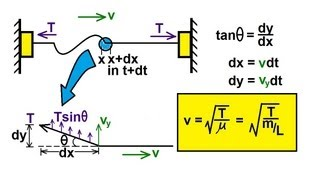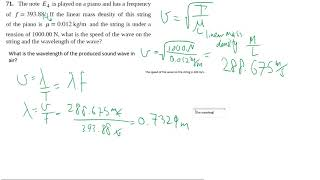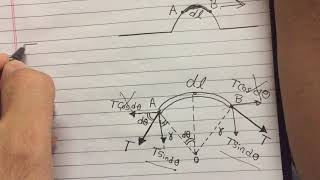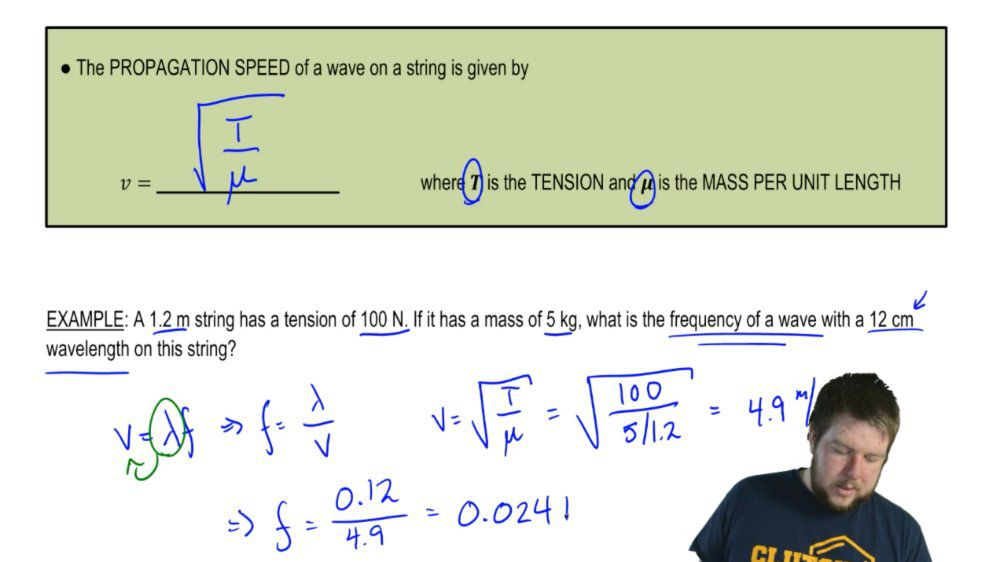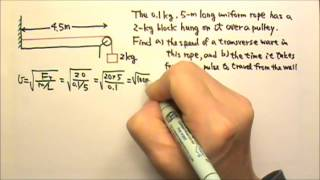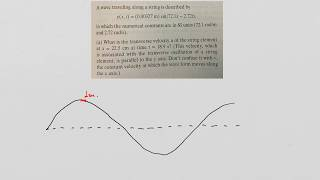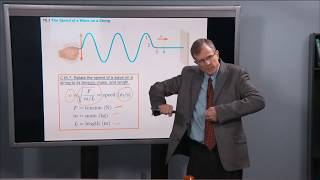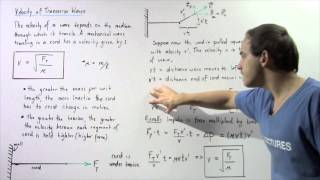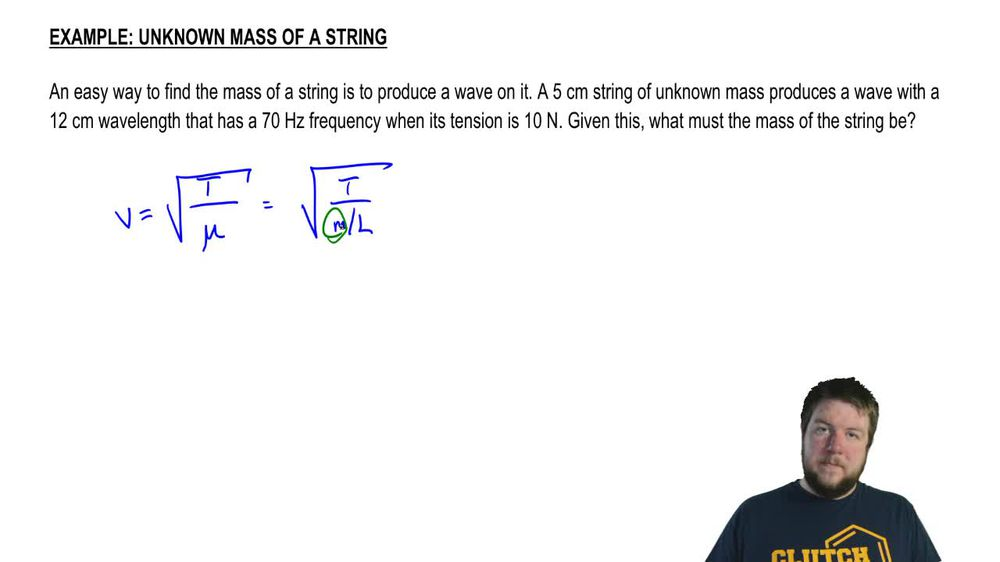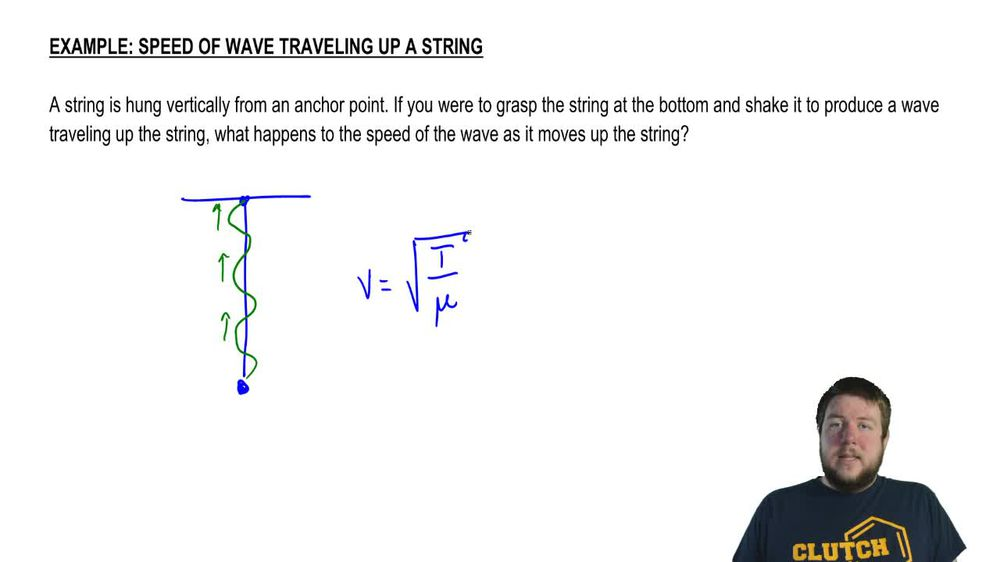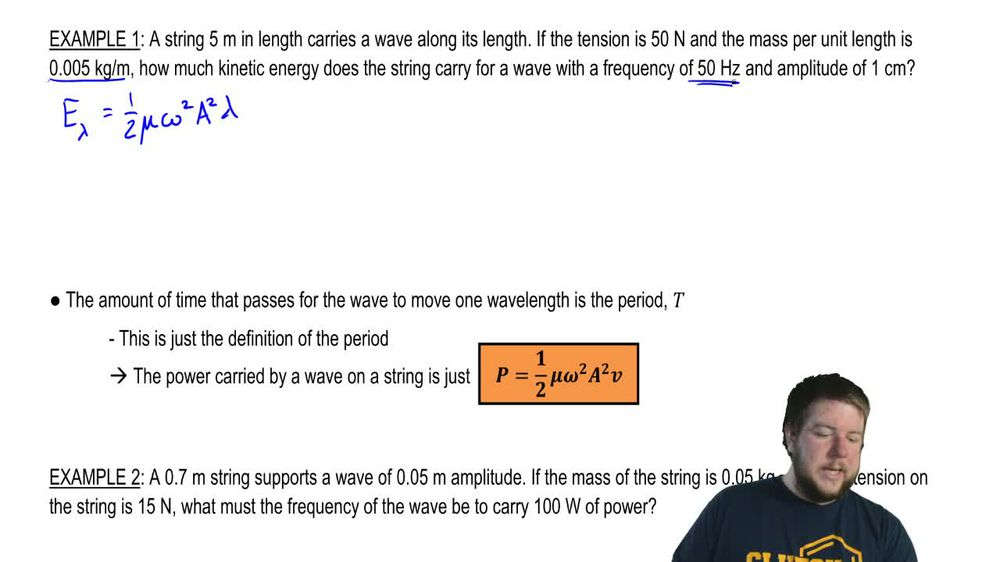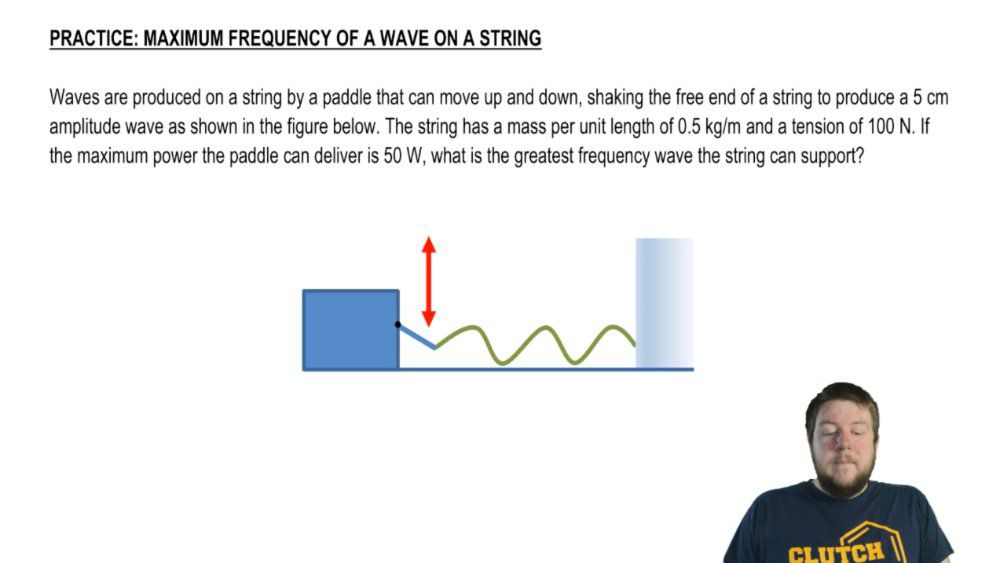Start typing, then use the up and down arrows to select an option from the list.
1. 18. Waves & Sound2. Velocity of Transverse Waves (Strings)# Waves On A String

by Patrick Ford
177 views
5
0
Hey, guys, in this video, we're gonna talk about waves on strings. Okay? If you were to fix a wave at one end against something like a wall, grab the other end and just whip it up and down, you would see that you produce a bunch of waves traveling down the length of that string. All right, so this is what we want to talk about. Now, as we've said before, a wave on a string is a very common example of a trans verse wave. One who's oscillations are perpendicular to its propagation. Okay, we explain why this was trance first before, but if you whip this string up and down, you're gonna produce these wave pulses that travel towards the wall. Okay, These waves that travel towards the wall now a wave on a string is no different, really than any other wave. In a lot of respects, it has the same mathematical description. It has the same characteristics on amplitude of propagation, speed, wavelength, frequency, etcetera. But there are a couple things that are unique to each wave. The specific energy carried by that wave is going to be unique to the wave and the specific propagation. Speed of that wave is gonna be unique to the wave. Remember that propagation speed depends upon two things. The type of wave and the characteristics of the medium that the wave is in. Okay, so what we're gonna focus ourselves on in this video is the speed of this wave. Okay? And of course, we're talking about the propagation speed. All right. The propagation speed of the wave on a string is given by the following equation. Spirit of tea over mu. Okay, where t is the tension in the string and mu? Is the mass per unit length of that strength? Okay, let's do a quick example. Ah, 1.2 m string as attention of 100 Newtons. If it has a massive 5 kg, what is the frequency of a wave with a 12 centimeter wavelength on this street? Okay, well, we know we want to find the frequency of the wave, okay? And we're told the wavelength. Since we are dealing with both frequency and wavelength, we're probably going to be using the equation. V equals Lambda F. And we can easily solve for F by dividing Lambda over. So the only question is What's the the speed on the string? Well, we know that for waves on a string, the speed is just the tension divided by the mass per unit length. Okay, we know the tension. We know the length and we know the mass. So we know everything we need to solve this problem. This is gonna be 100 Newtons of tension divided by the mass per unit length. So it's 5 kg and mass and it's 1.2 m in length. So that whole thing is going to be about 4.9 meters per second. Okay, This means that the frequency is 012 m. It was given in centimeters, but I need to convert 2 m divided by 4.9 m per second, which equals hurts. All right. No big deal. That wraps up our discussion on our introduction. Toe waves on the strength. Thanks for watching guys